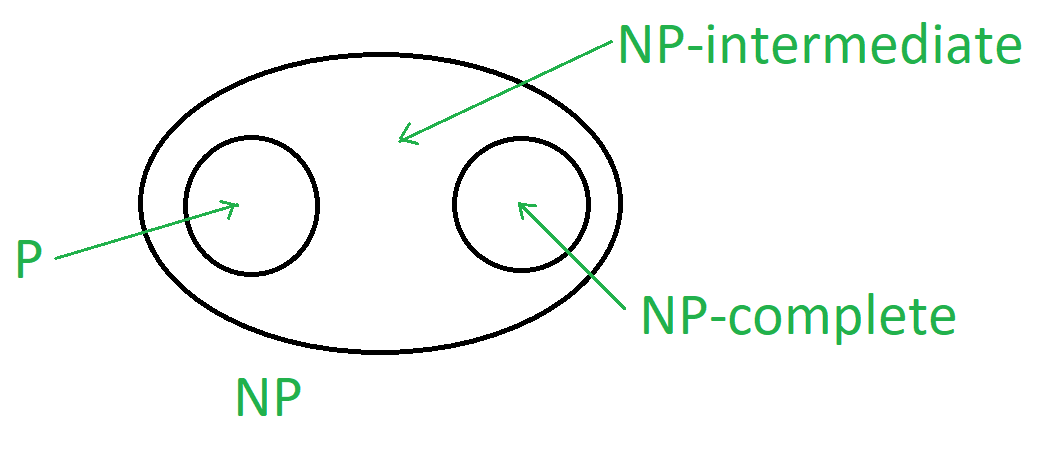Open in App
Not now

• Last Updated : 21 Apr, 2022

As you presumably know, regardless of whether  P = NP is a significant perplexing issue in field of Computer Science. In computational complexity, those problems which belongs to NP- problems but can’t belong to P or  NP-complete are known as NP-intermediate problems.

Taking into account NP-complete issues, it is normal to keep thinking about whether we have a division among P and NP-complete, to be specific whether  P or NP comprises of just NP-complete issues,  on the off chance that P  ≠ NP.

Even if you think of P  ≠ NP , it is enticing to believe that NP  =  P ∪ NP-complete – that each issue in NP can either be tackled in polynomial time or is sufficiently expressive to encode SAT. All this issues was solved by Ladner , his theorem proves that intermediate complexity exist.

NP-intermediate Problems :
A language L ∈ NP is NP-intermediate if and only if L∉ P and L∉ NP-complete.If P ≠ NP then there is a language L which is NP intermediate language.
In other words if P ≠ NP is true, then NP Intermediate is not empty, it means NP contains problems which are neither in P nor NP-complete.

Proof :
By using diagonalization,

let us assume a special function H : N –> N such that :

• O(m3) time required to process H(m) from m.
• H(m) –> ∞ with m if  SATH ∉ P.
• H(m) ≤ C (C= constant) if SATH ∈ P .
• Now,  Let SATH = {Ψ0 1 m^ H(m) : Ψ ∈ SAT and |Ψ| = m}

Taking P ≠ NP into account,  H is characterized so that  SATH  is NP-intermediate .

1. Let SATH ∈ P. Then H(m) ≤ C.
This suggests a poly-time algorithm for SAT as follows :

• first input ϕ , and get the value of m = |ϕ|.
• Now generate a string ϕ 0 1 m^ H(m) from calculated H(m).
• Verify if  string ϕ 0 1 m^ H(m)  ∈ SATH.

Result – Hence it should be SATH ∉ P , because of P ≠ NP.

2.  Let SATH ∈ NP-complete. This implies  H(m)–>∞ with m.
This suggests a poly-time algorithm for SAT as follows :

• SAT ≤p SATH   and  ϕ–> Ψ 0 1
• first input ϕ with m = |Ψ| , and get the value of f(ϕ) = Ψ 0 1k.
• Verify if k = m H(m) by processing H(m).
• This suggest , n c = |f(ϕ)| ≥ k ≥ m2c .

Hence, √n ≥ m Also ϕ ∈ SAT if  Ψ ∈ SAT.
Only O(log log n) recursive steps needed.

Result – Hence SATH ∉  NP-complete, as P ≠ NP.

Construction of H :
Now for H construction , we notice that the value of H(m) govern
membership on SATH of strings, Here length of SATH of string ≥ m.

• Hence we will define H(m) whose length is < m , according to string in SATH .
• Now for construction, we know that H(m) is the smallest k < log (log m) such that Mk  chooses participation of all length up to  log m strings x in SATH inside k. |x| k  time.    If not then we can say  H(m) = log (log m).
• Now H(m) ≤ C if and only if SATH ∈ P is true.

There is a poly-time M that chooses  enrollment of each x ∈ SATH inside c. |x| c  time.  Here M can be addressed by vastly many strings,  there’s α ≥ c such that M = M*α chooses participation of each x ∈ SATH inside α.|x| α  time.
Hence H(m) ≤ α for all m which satisfy α < log (log m).

• If SATH ∈ P then H(m) ≤ C (for infinitely many m).
H(m) = k for infinitely many m when  k ≤ C this condition true.

make any x ∈ {0,1}* now find largest M such that |x| ≤ log m and H(m) = k. Here x is decided by Mk in k. |x| k time.
SATH is determined by Mk which is poly-time machine.

Properties of H :
Properties of H are as follows :

• O(m3) time required to process H(m) from m.
• H(m) –> ∞ with m if  SATH ∉ P.
• H(m) ≤ C (C= constant) if SATH ∈ P .

Limits of diagonalization :
Diagonalization is a technique used to separate sets . Here we want to separate two sets NP and P for NP intermediate set. Kozen theorem shows that strong diagonalization does not relativize.

While the P versus NP question is still yet unsettled, we have refined our attention on the subject. Despite the fact that there is solid proof against diagonalization’s capacity to isolate P and NP, we have shown that it this doesn’t matter to our thought of solid diagonalization. Besides, solid diagonalization is the best way to isolate these P and NP, as Kozen illustrated in his theorem.

Examples of NP-intermediate problem :

• Computing the discrete logarithm.
• Graph isomorphism problem.
•  Factoring the discrete logarithm.
• Approximation of shortest vector in a lattice.
• Minimum Circuit Size Problem.
My Personal Notes arrow_drop_up